Question

# Calculating OCF. Ridiculousness, Inc. has sales of \$43,800, costs of \$22,700, depreciation expense of \$2,100, and...

Calculating OCF. Ridiculousness, Inc. has sales of \$43,800, costs of \$22,700, depreciation expense of \$2,100, and interest expense of 1,600. If the tax rate is 35%, what is the operating cash flow (OCF)? Show your Income Statement & computation.

Solution :

The Operating cash flow ( OCF ) = \$ 13,410.00

Please find the attached screenshot of the excel sheet containing the Income statement and a detailed computation / calculation for the solution.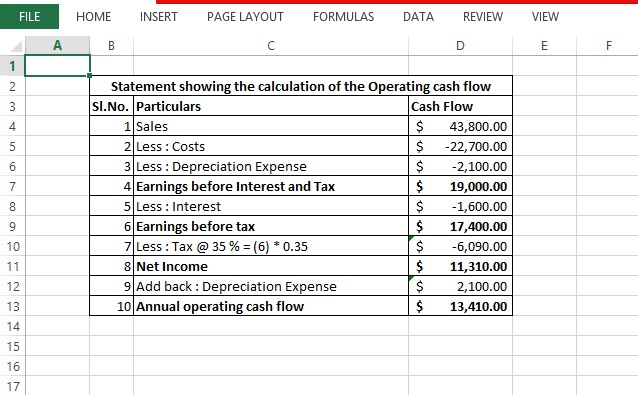#### Earn Coins

Coins can be redeemed for fabulous gifts.

Similar Homework Help Questions
• ### Fergus Inc. has sales of \$43,000, costs of \$19,100, depreciation expense of \$2,600, and interest expense...

Fergus Inc. has sales of \$43,000, costs of \$19,100, depreciation expense of \$2,600, and interest expense of \$2,100. If the tax rate is 35%, what is the operating cash flow, or OCF? (Omit \$ sign in your response.) Operating cash flow           \$

• ### Ridiculousness, Inc., has sales of \$43,500, costs of \$19,900, depreciation expense of \$1,600, and interest expense...

Ridiculousness, Inc., has sales of \$43,500, costs of \$19,900, depreciation expense of \$1,600, and interest expense of \$1,100. If the tax rate is 35 percent, what is the operating cash flow, or OCF? (Do not round intermediate calculations.) Operating cash flow \$

• ### Pompeli, Inc, has sales of \$46,500, costs of \$21,600, depreciation expense of \$1.90o, and interest expense...Pompeli, Inc, has sales of \$46,500, costs of \$21,600, depreciation expense of \$1.90o, and interest expense of \$1,650. If the tax rate is 21 percent, what is the operating cash flow, or OCF? (Do not round intermediate calculations.) Operating cash flow < Prev 80f 10 İİİ Next > e n e to search

• ### All answers must be answered as a formula 1. Calculate the operating cash flow Calculating OCF-Exce...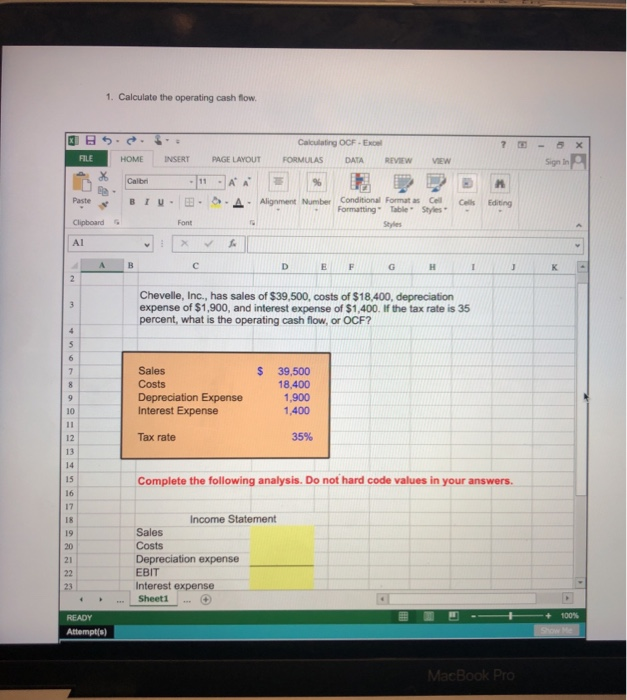All answers must be answered as a formula 1. Calculate the operating cash flow Calculating OCF-Exce HOME INSERTPAGE LAYOUTFORMULAS DATA REVIEWVEW Sign In Paste Clipboard Al T-3A Aligen berEatng Formatting Table Styles E F Chevelle, Inc., has sales of \$39,500, costs of \$18,400, depreciation expense of \$1,900, and interest expense of \$1,400. If the tax rate is 35 percent, what is the operating cash flow, or OCF? S 39,500 18.400 1,900 1,400 Sales Depreciation Expense Interest Expense 10 Tax rate...

• ### Pompeii, Inc., has sales of \$49,500, costs of \$22,800, depreciation expense of \$2,200, and interest expense...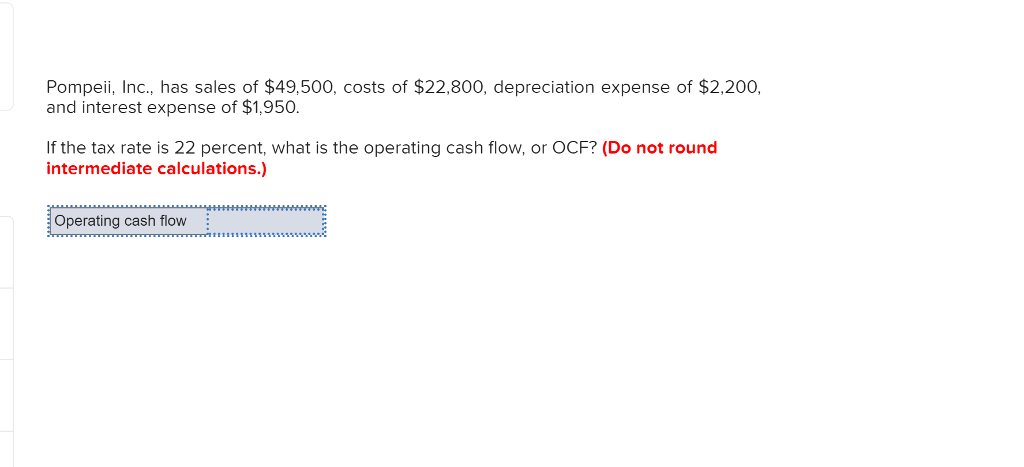Pompeii, Inc., has sales of \$49,500, costs of \$22,800, depreciation expense of \$2,200, and interest expense of \$1,950. If the tax rate is 22 percent, what is the operating cash flow, or OCF? (Do not round intermediate calculations.) Operating cash flow

• ### Pompeii, Inc., has sales of \$52,500, costs of \$24,000, depreciation expense of \$2,500 and interest expense...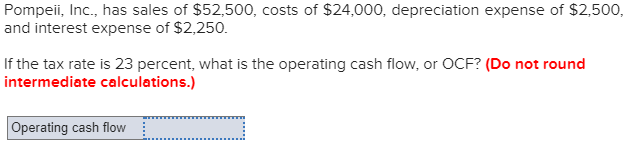Pompeii, Inc., has sales of \$52,500, costs of \$24,000, depreciation expense of \$2,500 and interest expense of \$2,250. If the tax rate is 23 percent, what is the operating cash flow, or OCF? (Do not round intermediate calculations.) Operating cash flow

• ### ompei, Inc., has sales of \$51,500, and interest expense of \$2150 costs of \$23.600, depreciation expense...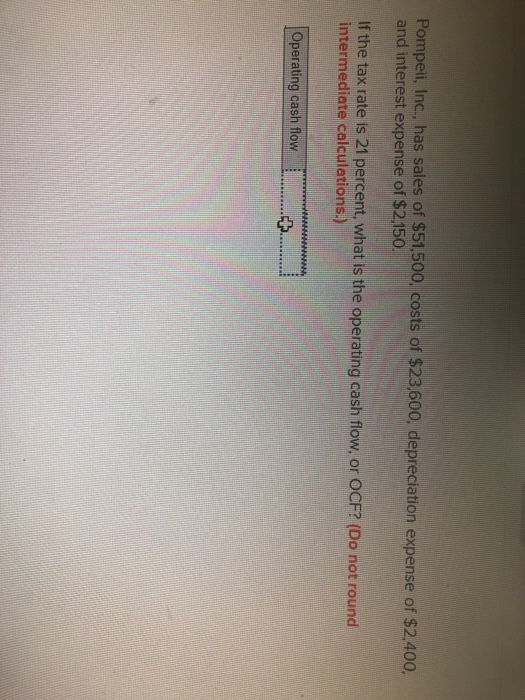ompei, Inc., has sales of \$51,500, and interest expense of \$2150 costs of \$23.600, depreciation expense of \$2,400. If the tax rate is 21 percent, what is the operating cash flow, or OCF? (Do not round intermediate calculations Operating cash flow :

• ### Pompeii, Inc., has sales of \$53,000, costs of \$24,200, depreciation expense of \$2,550, and interest expense...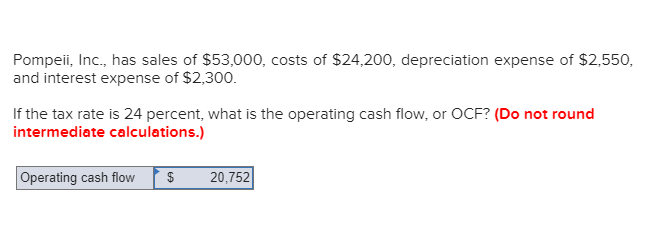Pompeii, Inc., has sales of \$53,000, costs of \$24,200, depreciation expense of \$2,550, and interest expense of \$2,300. If the tax rate is 24 percent, what is the operating cash flow, or OCF? (Do not round intermediate calculations.) Operating cash flow \$ 20,752||

• ### Chevelle, Inc. has sales of \$65978, costs of \$22683, depreciation expense of \$16002, and interest expense...

Chevelle, Inc. has sales of \$65978, costs of \$22683, depreciation expense of \$16002, and interest expense of \$2744. If the tax rate is 33 percent, what is the operating cash flow, or OCF?

• ### Hammett, Inc., has sales of \$69,543, costs of \$34,857, depreciation expense of \$14,381, and interest expense...

Hammett, Inc., has sales of \$69,543, costs of \$34,857, depreciation expense of \$14,381, and interest expense of \$3,504. If the tax rate is 25 percent, what is the operating cash flow, or OCF?